Have a question? Connect with the community at the TensorFlow Forum

# Adversarial example using FGSM

This tutorial creates an adversarial example using the Fast Gradient Signed Method (FGSM) attack as described in Explaining and Harnessing Adversarial Examples by Goodfellow et al. This was one of the first and most popular attacks to fool a neural network.

## What is an adversarial example?

Adversarial examples are specialised inputs created with the purpose of confusing a neural network, resulting in the misclassification of a given input. These notorious inputs are indistinguishable to the human eye, but cause the network to fail to identify the contents of the image. There are several types of such attacks, however, here the focus is on the fast gradient sign method attack, which is a white box attack whose goal is to ensure misclassification. A white box attack is where the attacker has complete access to the model being attacked. One of the most famous examples of an adversarial image shown below is taken from the aforementioned paper.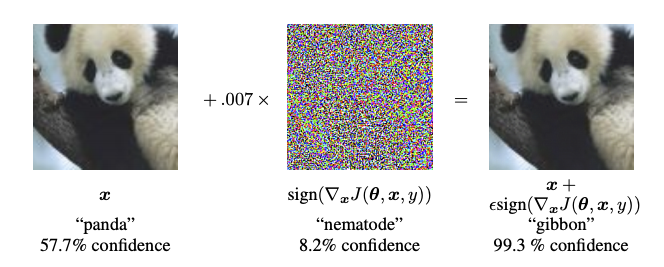Here, starting with the image of a panda, the attacker adds small perturbations (distortions) to the original image, which results in the model labelling this image as a gibbon, with high confidence. The process of adding these perturbations is explained below.

## Fast gradient sign method

The fast gradient sign method works by using the gradients of the neural network to create an adversarial example. For an input image, the method uses the gradients of the loss with respect to the input image to create a new image that maximises the loss. This new image is called the adversarial image. This can be summarised using the following expression:

$$adv\_x = x + \epsilon*\text{sign}(\nabla_xJ(\theta, x, y))$$

where

• adv_x : Adversarial image.
• x : Original input image.
• y : Original input label.
• $\epsilon$ : Multiplier to ensure the perturbations are small.
• $\theta$ : Model parameters.
• $J$ : Loss.

An intriguing property here, is the fact that the gradients are taken with respect to the input image. This is done because the objective is to create an image that maximises the loss. A method to accomplish this is to find how much each pixel in the image contributes to the loss value, and add a perturbation accordingly. This works pretty fast because it is easy to find how each input pixel contributes to the loss by using the chain rule and finding the required gradients. Hence, the gradients are taken with respect to the image. In addition, since the model is no longer being trained (thus the gradient is not taken with respect to the trainable variables, i.e., the model parameters), and so the model parameters remain constant. The only goal is to fool an already trained model.

So let's try and fool a pretrained model. In this tutorial, the model is MobileNetV2 model, pretrained on ImageNet.

import tensorflow as tf
import matplotlib as mpl
import matplotlib.pyplot as plt

mpl.rcParams['figure.figsize'] = (8, 8)
mpl.rcParams['axes.grid'] = False


Let's load the pretrained MobileNetV2 model and the ImageNet class names.

pretrained_model = tf.keras.applications.MobileNetV2(include_top=True,
weights='imagenet')
pretrained_model.trainable = False

# ImageNet labels
decode_predictions = tf.keras.applications.mobilenet_v2.decode_predictions

Downloading data from https://storage.googleapis.com/tensorflow/keras-applications/mobilenet_v2/mobilenet_v2_weights_tf_dim_ordering_tf_kernels_1.0_224.h5
14540800/14536120 [==============================] - 1s 0us/step

# Helper function to preprocess the image so that it can be inputted in MobileNetV2
def preprocess(image):
image = tf.cast(image, tf.float32)
image = tf.image.resize(image, (224, 224))
image = tf.keras.applications.mobilenet_v2.preprocess_input(image)
image = image[None, ...]
return image

# Helper function to extract labels from probability vector
def get_imagenet_label(probs):
return decode_predictions(probs, top=1)


## Original image

Let's use a sample image of a Labrador Retriever by Mirko CC-BY-SA 3.0 from Wikimedia Common and create adversarial examples from it. The first step is to preprocess it so that it can be fed as an input to the MobileNetV2 model.

image_path = tf.keras.utils.get_file('YellowLabradorLooking_new.jpg', 'https://storage.googleapis.com/download.tensorflow.org/example_images/YellowLabradorLooking_new.jpg')
image_raw = tf.io.read_file(image_path)
image = tf.image.decode_image(image_raw)

image = preprocess(image)
image_probs = pretrained_model.predict(image)

Downloading data from https://storage.googleapis.com/download.tensorflow.org/example_images/YellowLabradorLooking_new.jpg
90112/83281 [================================] - 0s 0us/step


Let's have a look at the image.

plt.figure()
plt.imshow(image * 0.5 + 0.5)  # To change [-1, 1] to [0,1]
_, image_class, class_confidence = get_imagenet_label(image_probs)
plt.title('{} : {:.2f}% Confidence'.format(image_class, class_confidence*100))
plt.show()

Downloading data from https://storage.googleapis.com/download.tensorflow.org/data/imagenet_class_index.json
40960/35363 [==================================] - 0s 0us/step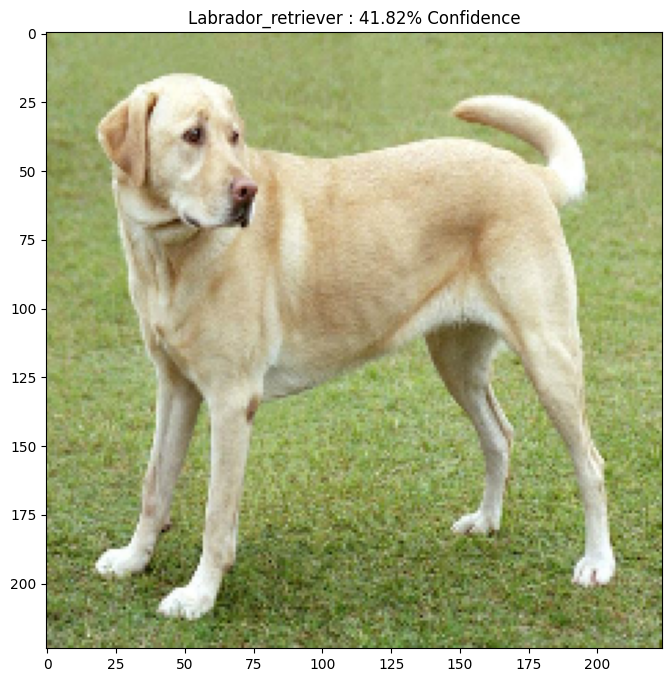## Create the adversarial image

### Implementing fast gradient sign method

The first step is to create perturbations which will be used to distort the original image resulting in an adversarial image. As mentioned, for this task, the gradients are taken with respect to the image.

loss_object = tf.keras.losses.CategoricalCrossentropy()

def create_adversarial_pattern(input_image, input_label):
with tf.GradientTape() as tape:
tape.watch(input_image)
prediction = pretrained_model(input_image)
loss = loss_object(input_label, prediction)

# Get the gradients of the loss w.r.t to the input image.
gradient = tape.gradient(loss, input_image)
# Get the sign of the gradients to create the perturbation
signed_grad = tf.sign(gradient)
return signed_grad


The resulting perturbations can also be visualised.

# Get the input label of the image.
labrador_retriever_index = 208
label = tf.one_hot(labrador_retriever_index, image_probs.shape[-1])
label = tf.reshape(label, (1, image_probs.shape[-1]))

perturbations = create_adversarial_pattern(image, label)
plt.imshow(perturbations * 0.5 + 0.5);  # To change [-1, 1] to [0,1]

<matplotlib.image.AxesImage at 0x7f4171d66690>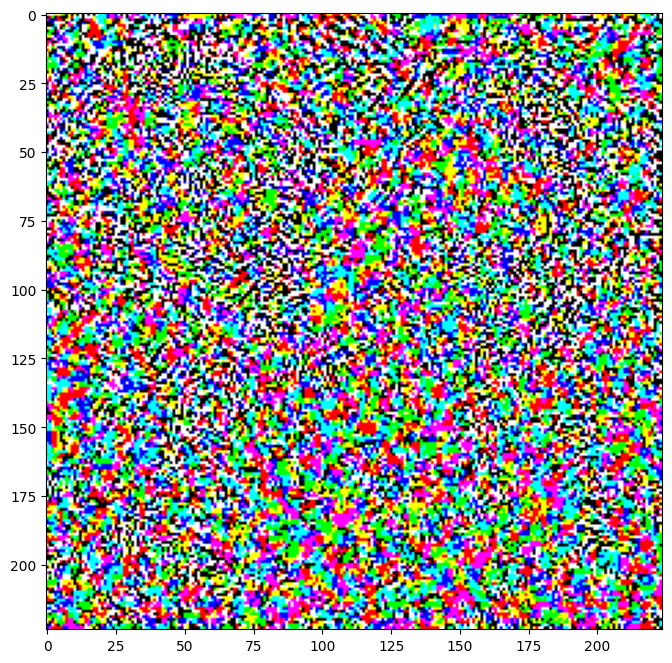Let's try this out for different values of epsilon and observe the resultant image. You'll notice that as the value of epsilon is increased, it becomes easier to fool the network. However, this comes as a trade-off which results in the perturbations becoming more identifiable.

def display_images(image, description):
_, label, confidence = get_imagenet_label(pretrained_model.predict(image))
plt.figure()
plt.imshow(image*0.5+0.5)
plt.title('{} \n {} : {:.2f}% Confidence'.format(description,
label, confidence*100))
plt.show()

epsilons = [0, 0.01, 0.1, 0.15]
descriptions = [('Epsilon = {:0.3f}'.format(eps) if eps else 'Input')
for eps in epsilons]

for i, eps in enumerate(epsilons):
adv_x = image + eps*perturbations
adv_x = tf.clip_by_value(adv_x, -1, 1)
display_images(adv_x, descriptions[i])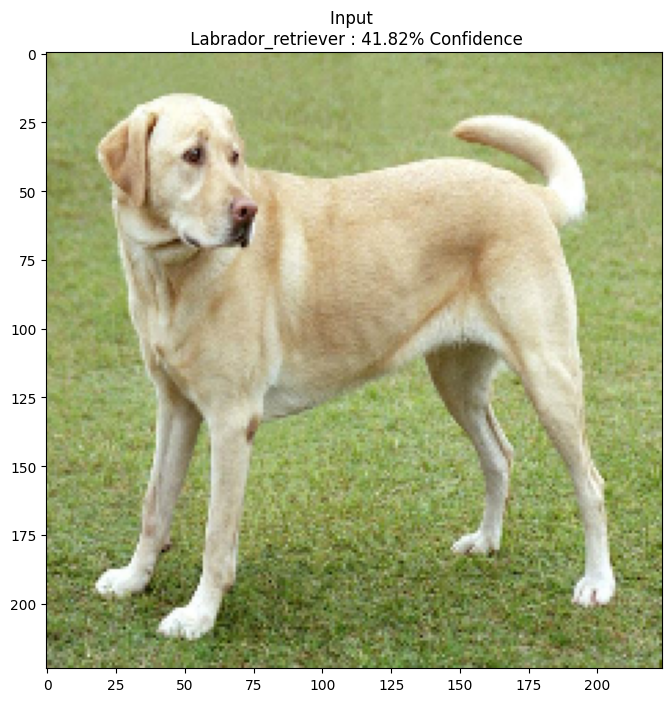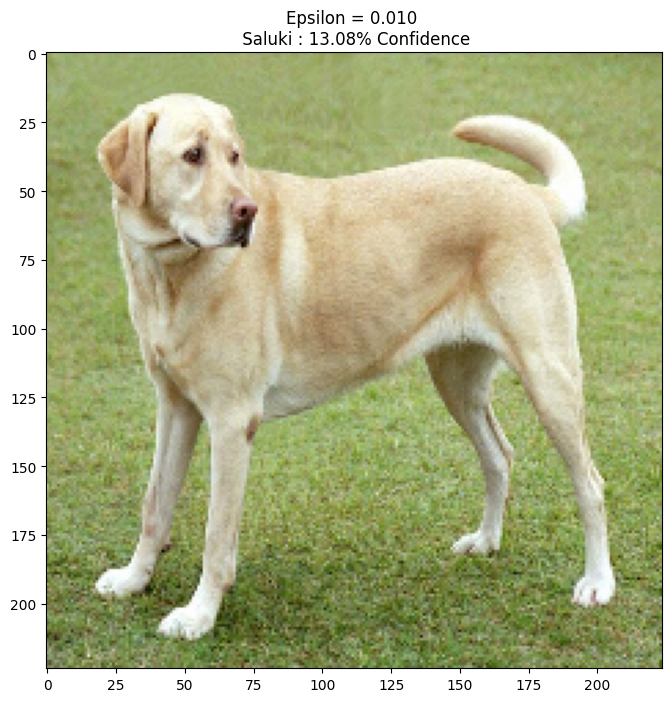## Next steps

Now that you know about adversarial attacks, try this out on different datasets and different architectures. You may also create and train your own model, and then attempt to fool it using the same method. You can also try and see how the confidence in predictions vary as you change epsilon.

Though powerful, the attack shown in this tutorial was just the start of research into adversarial attacks, and there have been multiple papers creating more powerful attacks since then. In addition to adversarial attacks, research has also led to the creation of defenses, which aims at creating robust machine learning models. You may review this survey paper for a comprehensive list of adversarial attacks and defences.

For many more implementations of adversarial attacks and defenses, you may want to see the adversarial example library CleverHans.

[{ "type": "thumb-down", "id": "missingTheInformationINeed", "label":"Missing the information I need" },{ "type": "thumb-down", "id": "tooComplicatedTooManySteps", "label":"Too complicated / too many steps" },{ "type": "thumb-down", "id": "outOfDate", "label":"Out of date" },{ "type": "thumb-down", "id": "samplesCodeIssue", "label":"Samples / code issue" },{ "type": "thumb-down", "id": "otherDown", "label":"Other" }]
[{ "type": "thumb-up", "id": "easyToUnderstand", "label":"Easy to understand" },{ "type": "thumb-up", "id": "solvedMyProblem", "label":"Solved my problem" },{ "type": "thumb-up", "id": "otherUp", "label":"Other" }]